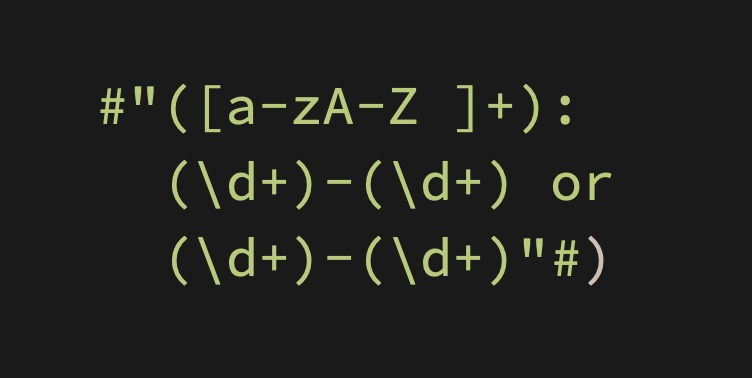# Dimitar Chakarov# Regular Expressions FTW

January 17, 2021 | 3 Minute Read

When I started doing the Advent of Code 2020 challenges in December I thought I can get away with not using regular expressions. I tried relying on string manipulation alone. I wrote a whole bunch of `replacingOccurrences(of:` and `components(separatedBy:`. It was all well and good until I reached Day 4. I managed to figure out how to do most of the parsing without regular expressions but the height bit was tough. The rules were:

``````hgt (Height) - a number followed by either cm or in:
If cm, the number must be at least 150 and at most 193.
If in, the number must be at least 59 and at most 76.
``````

After some googling, coding and debugging I had the following solution:

``````func heightValid(_ height: String) -> Bool {
let pattern = #"(\d{2,3})(cm|in)"#
let regex = try! NSRegularExpression(pattern: pattern, options: [])
let nsrange = NSRange(height.startIndex..<height.endIndex, in: height)
var valid = false
regex.enumerateMatches(in: height,
options: [],
range: nsrange) { (match, _, stop) in
guard let match = match else { return }
if match.numberOfRanges == 3,
let firstCaptureRange = Range(match.range(at: 1), in: height),
let secondCaptureRange = Range(match.range(at: 2), in: height) {
let number = Int(height[firstCaptureRange])!
let metric = String(height[secondCaptureRange])
if metric == "cm" {
if number >= 150 && number <= 193 { valid = true }
} else if metric == "in" {
if number >= 59 && number <= 76 { valid = true }
}
stop.pointee = true
}
}
return valid
}
``````

It was working great. The only challenge I had was that it was a bit lengthy. If I was to use this approach for the next challenges I needed it to be more modular.

I decided to create a framework that gives me a generalised version of this method which I would be able to use going forward. I didn’t want to introduce third party dependency managers so I opted for SPM. My goal was to be able to write as little code as possible at the caller side. Here is what I came up with:

``````let helper = RegexHelper(pattern: #"(\d{2,3})(cm|in)"#)
let results = helper.parse(inputString)
``````

Making the framework open source was a no brainer. You can get it here. Any questions or suggestions? Ping me on Twitter or raise a pull request.